# Rubik's Slide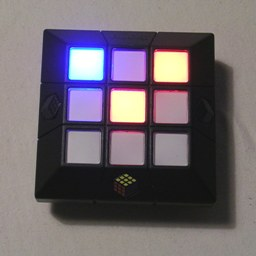Rubik's Slide is an electronic puzzle played on a 3×3 grid of lights. The top part of the puzzle containing the grid of lights can be pushed left, right, up, or down, and this causes the pattern of lights to shift one step in that direction. Any lights on the furthest edge that would fall off the grid reappear on the opposite edge of the grid. The grid can also be rotated in either direction, and depending on the difficulty level, either causes the pattern to rotate by 90 degrees, or causes the eight outer lights to shift along cyclically. A random goal pattern is generated, and your aim is to reach that pattern from the starting pattern which is also randomly generated.

Apart from the moving top section, there are three buttons.

SymbolMeaningExplanation
EqualsScoreSpeaks the current score
TickGoalDisplays the goal pattern
TrianglePlayStart play, or restart this level.

The off switch on the back of the puzzle has two on-settings. In the middle setting (marked by the infinity symbol) you have free play, i.e. without time constraints. The rightmost setting (marked by a clock symbol) is the lightning round, where you play ten puzzles each with a time limit.

There are three types of difficulty for the puzzles.
1. The twisting move does a quarter turn. One to four lights of one or two colours are used.
2. The twisting move cycles the 8 outer lights. Two to five lights of only one colour are used.
3. The twisting move cycles the 8 outer lights. Two to six lights of one or two colours are used. In free play mode, you only play one of these types of puzzle, as given by the difficulty setting. In lightning round mode all three types of puzzle occur, but the time limit you are given depends on both the difficulty of the particular puzzle and the overall difficulty setting.

Selecting difficulty: When you switch on the puzzle it will show a little animation, and then you can select the difficulty level by pushing the grid up or down to light up one, two, or three rows. You can hear your best score by pressing the score button, or start play by pressing the Play button. You can re-select the difficulty level during play by holding down the Play and Score buttons simultaneously for a few seconds.

Selecting volume: The volume setting can be accessed by holding down the Goal and Play buttons simultaneously for a few seconds. The volume setting can then be changed by turning the grid clockwise for louder, anti-clockwise for quieter. At the lowest setting the sound is switched off. To exit the volume display, just wait a few seconds without changing the volume level.

Resetting: You can completely reset the puzzle so that it forgets all the scores by holding down the Score and Goal buttons simultaneously for a five seconds, and then also pressing the Play button at the same time.

If your browser supports it, you can click on the link below to see a Javascript version of the Rubik's Slide. It does not do level 1 quarter turn puzzles, only the level 2/3 puzzles with 8-cycle moves.

## The number of positions:

When playing a puzzle of difficulty type 1, you can only do shifts and quarter turns. If the light pattern has no symmetry, this leads to 9×4 = 36 different patterns. If the pattern does have some kind of symmetry, then there are 6, 9 or 18 possible patterns you can reach.

Puzzle of the other types difficulty are much more interesting. If you think of the lights as having 9 different colours, then using shifts and the 8-cycle turns all 9! = 362,880 permutations of these lights is possible. So given any two patterns that for each colour have the same number of lights, they can be transformed into one another. The number of patterns for a given number of lights is listed in this table:

12345
093684126126
172252504630
27561,260
31,680

I have only given the numbers for those combinations that are actually produced by the puzzle. The numbers are calculated using the formula 9! / (a! b! (9-a-b)! ).

## Solution:

### Level 1:

Puzzle of this difficulty level are quite easy, as there are at most 36 patterns you can reach using slide moves and quarter turns. Imagine the 3×3 pattern of lights is repeated in all directions like bathroom tiles. The 3×3 pattern you see is like a window onto this tiling pattern. Slide moves and quarter turns simply shift or turn the window through which you look at this tiling. Take a look at the following tilings: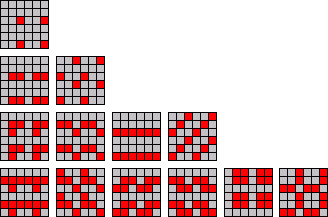Every possible 3×3 pattern is represented somewhere, in some orientation. For patterns with 5 lights on, you will have to use the tilings with 4 lights (the bottom row) and just concentrate on solving the four unlit squares instead of the five lit ones. If two colours of lights are used, it actually tends to be easier. If one colour has two lights, then you can first solve only those two lights. If this has not solved the whole pattern, then you must swap those two lights by turning 180 degrees, and sliding them back into place. Similarly if one colour has three lights, solve those first. If they lie in one of the left two patterns in the picture above (row 3) then it will be solved. If one of the other two patterns, then they lie in a line and you must slide along that line until it is solved. In every case you just solve the (partial) pattern, and if the pattern has some symmetry then apply those symmetries to get variants of that same (partial) pattern. Instead of just ignoring one colour completely you may also just treat all lights as if they were the same colour, or only concentrate on the unlit squares as the third colour. Puzzles of this difficulty level soon become very easy.

### Level 2:

If there are only two lights on, then there are 36 patterns, or only 10 if patterns which differ by a quarter turn are considered the same. This graph shows their relationships: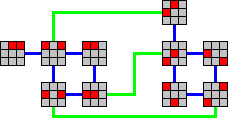Blue lines are slide moves, the green lines represent turn moves (8-cycle). There are two blocks of patterns - those where the two lights are adjacent horizontally or vertically (where opposite edges of the grid are considered adjacent), and those where they are adjacent diagonally.

With three lights the patterns are grouped into four blocks.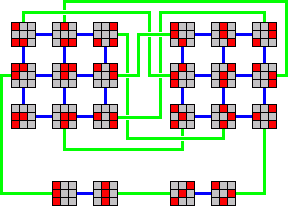Finally the patterns with four lights are grouped into six blocks.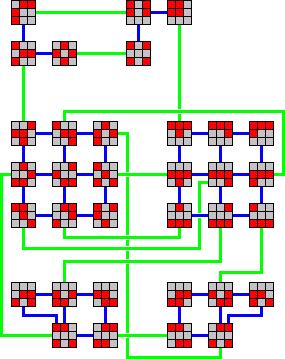For patterns with five lights you can use the graph above of four-light patterns and apply it to the four unlit squares of the puzzle.

## Notation:

Let U, D, L, R denote slide moves in the directions Up, Down, Left, and Right. A clockwise or anti-clockwise turn move is denoted by C or A respectively.

### Level 3:

Using the techniques above you can usually solve part of the pattern, leaving only a few lights to be solved. Here are a few move sequences that move only a few lights without disturbing the rest.

 Swap top left and top middle pieces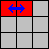UADA UADA UAD CRC Swap top left and centre pieces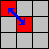CRAL CRAL CUCUC RD Slide only middle row to the left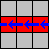CLUAL CLUAL Cycle left, top left, and top pieces.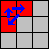DARUAL DARUAL

To swap any two pieces, use slide moves to put the two pieces into locations matching those of either of the first two sequences above. You may need to hold the puzzle a different way up for that to be possible. Apply the appropriate sequence to swap them, and then undo the set-up slide moves bringing the pieces back to where they started.

Another method is to take advantage of the fact that some patterns with one colour lights are very symmetric. For example, if two reds and a blue lie in a horizontal row then you can slide it left or right to make any one of the lights blue. If you know which light goes where as you transform it to any other three-light shape, then you can solve any three-light pattern regardless of the colours. The following graph helps with this.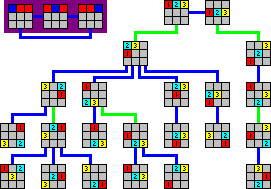So you can solve it as follows:
1. Hold the puzzle such that the current pattern matches one in the graph.
2. Follow the graph upwards to transform your pattern into three in a row.
3. Find the target pattern in the graph (ignoring orientation).
4. Note which of the lights in the your target pattern is unique in colour.
5. Use the numbers/colours in the graph to see which light that corresponds to in the three-in-a-row pattern.
6. Slide the three-in-a-row pattern to put the unique colour light in the right spot.
7. Follow the graph down to the target pattern.
8. If necessary rotate the pattern a quarter or a half turn to get it in the correct orientation.

The most symmetric patterns with four lights have the four corners lit, or the four edges lit. If you have a colour with only one light then you can easily rotate the symmetric pattern to get it to the right spot. If you have two of each colour, then you need to be able to swap between the two distinct cases (two lights of one colour are adjacent or opposite corners/edges). See the graph below.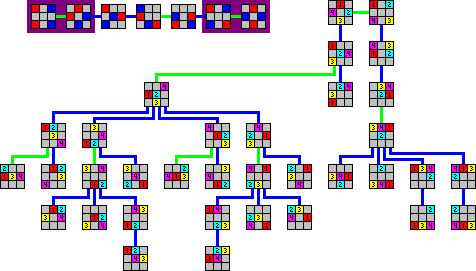For patterns with 5 lights we get a similar graph, but now there are more cases to consider. In the 4+1 colour distribution there are two cases (corner/edge or centre) and in the 3+2 distribution there are three (adjacent, opposite, or one in centre).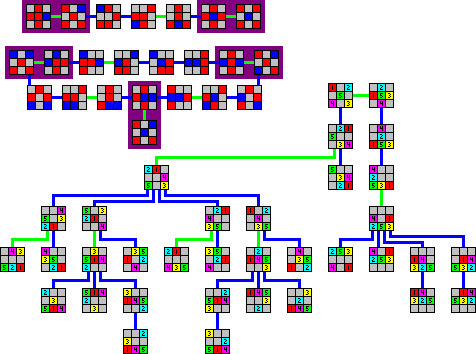There is no particularly symmetric pattern with six lights, so this technique breaks down there.